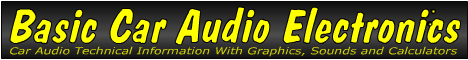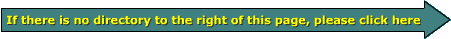Email   Home Pagex

 Web www.bcae1.com www.bcot1.com
Use F11 to go to full screen
Translate.

 AC Voltage vs DC Voltage All voltages and currents that we have discussed so far have been of the DC variety. The next section will describe the difference between AC and DC voltages. Direct Current: Direct current (DC) is the type of current that is supplied by batteries. The electrical force exerted by the battery is constant. This does not mean that it never changes, but it is not constantly changing polarity. The "difference of potential" (means the same as voltage) stays either positive or negative with respect to its "reference" (usually designated as ground). Alternating Current: Alternating current is different from DC in that it "periodically" changes polarity. "Periodically" in the United States is 120 times/second. This is 60 complete cycles per second. Different ways to express the amplitude/magnitude of an AC waveform will be covered on an upcoming page.If you look at the diagram above you will see that the DC voltage is constant. The AC waveform (a sine wave in this example) is continuously changing. Let's say that the voltage of "Z" is 10 volts DC. The AC waveform has a few points on it marked. The instantaneous voltage at those points are listed below. Please understand that the voltages are only at that point in time. If the waveform voltage was measured with a volt meter, it would read approximately 10 volts AC. All voltages are referenced to the black line. A. 11 volts B. 2 volts C. 5 volts D. -5 volts E. -13 volts

 You should remember: 1.D.C. is a type of voltage which does NOT cross a point of reference at regular intervals. 2.A.C. is a type of voltage which DOES cross a point of reference at regular intervals.

```

```